Electronic Structure and Magnetic Property in Strongly Correlated Material Systems (2) : Introduction to Mott Insulator and Magnetism

Viewed : 79 times,  2022-01-06 08:13:12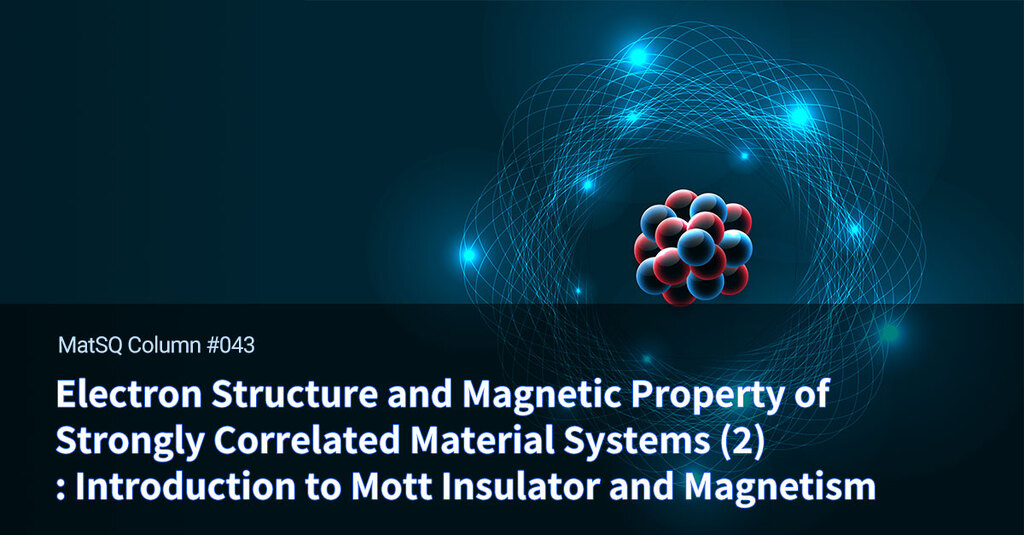(Continued from the previous column) In the last column, I briefly described the concepts of electronic correlation in condensed matter systems and why it can remain hidden in several electronic systems such as metals. In this column, the so-called “Mott insulator” state and “Mott–Hubbard magnetism” are introduced, which are most famous examples of correlation-induced phenomena occurring when the Coulomb interactions are poorly screened.

Comparison of transition metal oxide and metallic material

Materials showing a strong screening effect and weak electron–electron correlations include alkali metals, such as lithium and sodium, and metallic materials, such as aluminum, copper, silver, and gold. Meanwhile, transition metal / rare-earth metal oxides are the most representative materials that show electron correlations well (transition metal oxides). The commonality of metallic materials with weak correlation is that free electrons are distributed in s-orbitals located at the highest energy in metallic atoms, while in strongly correlated materials partially-filled d-orbitals in the transition metal ions oxide are where electron degrees of freedom are active. It means that most of physical properties of transition metal oxides, such as the conductivity and magnetism, are derived from the partially-filled d-orbitals.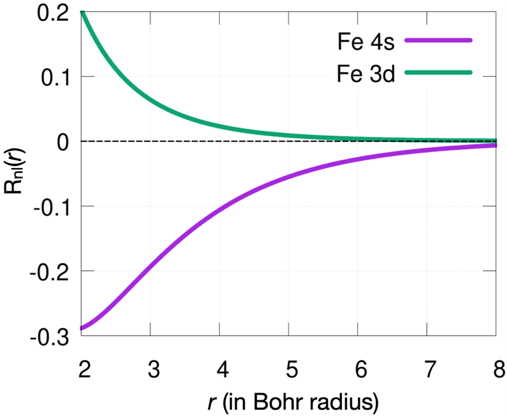Figure 1. The magnitude of radial wave function Rnl(r) according to distance from the atomic center of 3d- and 4s-orbitals in an iron atom. The wave function is derived from the potential database of OpenMX, the computational calculation code of density functional theory(https://t-ozaki.issp.u-tokyo.ac.jp/vps_pao2019/Fe/Fe_Hard/index.html).

[Figure 1] shows the radial distribution of outermost 4s- and 3d-orbitals in the of an iron atom, as a function of distance from the nucleus. It shows that the 4s-orbital is distributed farther than the 3d-orbital. The “hopping integral” between orbitals located at adjacent iron atoms, which is the magnitude of kinetic energy between adjacent atoms, can be calculated as follows.

$$t_{i,j} \equiv \left\langle \phi_i \begin{vmatrix} - {\hbar^2 \over 2m} \nabla ^2\end{vmatrix} \phi_i \right\rangle$$

Here, ϕi,j  is the orbital wavefunction of the atom site i and j. The magnitude tij , the kinetic energy between orbitals ϕi,j , is stronger as ϕi,j  are widely spread over space. That is, in the case of elemental iron metals containing outermost electrons in the 4s-orbital, it can be inferred that the screening effect and metallic property will occur as a result of strong electron kinetic energy. However, in the case of iron oxide where valence electrons are located in 3d-orbitals (some of electrons are occupied by covalent bonding with oxygen and, thus, have valence electrons in the 3d-orbital), it can be inferred that it has smaller kinetic energy, and its electron correlation becomes stronger.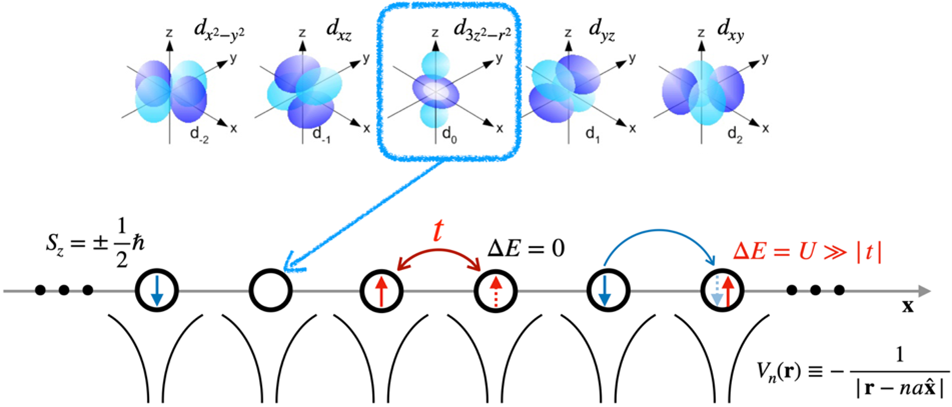Figure 2. Schematic figure showing a one-dimensional problem consisting only of d_{3z2-r2} orbitals (other d-orbitals are completely filled up with electrons or completely empty, so they do not affect conductivity) among the d-orbitals of a transition metal and energy difference when electrons transfer to an empty orbital and an orbital where electrons already existed

Introduction to Mott insulator through tight-binding model

Considering that most electrons are tied around atoms like transition metal oxides and electrons “jump” across adjacent atoms, the electron’s wave function can be represented employing localized wave functions around the atoms as the basis. It is called “tight-binding model”, which is often very convenient for explaining electronic properties of crystalline systems.

To simplify the problem, let us consider a one-dimensional chain system that consists only of d3z2-r2 orbitals of transition metal ions, and the hopping constant between 3dz2-r2 orbitals of adjacent atoms is given as (see Figure 2). In addition, suppose that the Coulomb interaction between electrons occurs only when two electrons are simultaneously occupying the same site (i.e., two electrons must have opposite spin Sz values by the Pauli exclusion principle). We denote the magnitude of this as U.

The physical properties of this system is determined by the following two parameters, a) t/U and b) the average number of electrons per orbital, n. t/U is the ratio between the Coulombic potential energy and kinetic energy of an electron in this problem; t/U being 0 or infinity correspond to situations where Coulombic potential energy or kinetic energy dominates over the other, respectively.

We skip the discussion on the weakly correlated t/U→∞ situation. Consider the situation where t/U is zero, which is the opposite limit. (It does not mean that the hopping constant t is zero. Rather, U is relatively much larger than t.) Even in this situation (see Figure 2), it can be found that electrons can mostly hop to the next orbital without an energy cost close to n=0, so I will exclude them from the discussion.

Exactly at n=1, when there exist the same number of electrons and orbitals, interesting states occur. In this case, energy is the lowest when every orbital is occupied by an electron, and transferring an electron to the next orbital requires not only satisfying the spin condition according to the exclusion principle but also having a very high energy cost of U (>> t). Therefore, unless energy is directly supplied from the external system, the electrons remain fixed in their initial position, so that electron transfer cannot occur and system becomes an insulator.

Because the arrangement of adjacent electrons strongly determines electron transport because of the presence of Coulomb interaction, it is an example of a strongly correlated electron system, and a state that becomes an insulator through an electron correlation is called the “Mott insulator state.” Therefore, the physics behind the Mott-insulating phase is difficult to be captured within a simple band theory picture.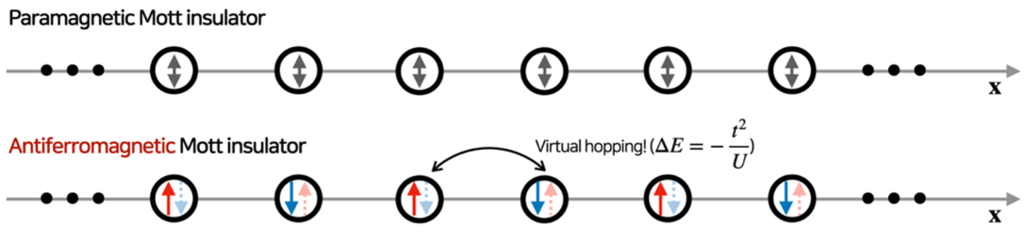Figure 3. Schematic figure of difference between paramagnetic/antiferromagnetic Mott insulator and generation of exchange interaction

Principles of magnetism exchange interaction and antiferromagnetism generation

The situation becomes more interesting when quantum fluctuations of electrons are considered in the above Mott insulator state. The electrons can virtually “hop to the next position and come back” thanks to the quantum fluctuation effect. It is denoted a virtual excitation by quantum fluctuation; therefore, the difference occurs between i) a state where electrons can “virtually” move to the next orbital and return, and ii) a state where virtual excitation is forbidden by the Pauli exclusion principle.

Let’s take a closer look at [Figure 3]. Suppose that a single electron is in each orbital in one dimension (e.g., d3z2-r2 orbital, etc.), as shown in [Figure 2], and the spin state (Sz = ±1/2) is random (i.e., a paramagnetic state). Here, if two electrons with different spins are adjacent to each other, the probability of electrons transferring from an existing orbital to another is very small if the Coulombic potential U can be overcome. Using the perturbation theory, it is known that the energy of two electrons can be lowered to $${t^2 \over U}$$ through this virtual excited state.

Meanwhile, among electrons with the same spin adjacent to each other, the possibility of an electron transferring even in a virtual system is blocked by the exclusion principle. You might think that electrons can be transferred if they hop into the next orbital and reverse the spin, but this would not happen unless there are external factors that could change the spin angular momentum, such as an external magnetic field. To sum it up:

• The case of electron spins at adjacent i, j orbitals are in the same direction: E=0• The case of electron spins at adjacent i, j orbitals are in opposite directions: energy gain of $$E = - {t^2\over U}$$ per a atomic pair occurs.

Then, the spin of electrons at i and j is Si,j, and the energy of the corresponding one-dimensional electron system can be written as follows.

$$E = J \sum\limits_{\left\langle ij \right\rangle}^{ } S_iS_j$$

Here, <i,j> means addition only when i, j are adjacent to each other, and then it would be $$J = - { 2t^2\over U}$$. Replacing Si,j into spin operators results in effective quantum spin Hamiltonian to the corresponding problem.

As such, the interaction J among spins generated by the virtual excitations in the Mott insulator is called “magnetic exchange interactions,” which results in a variety of magnets in daily life. Furthermore, in the above example, the exchange interaction causes adjacent electrons to preferably show opposite spins, and the state where spin magnetic dipoles of a material are aligned in opposite directions is called an “antiferromagnetism” state.

Finally, the above explanation does not consider the effect of thermal fluctuation from the thermal equilibrium on the external environment. Therefore, the argument given above applies at a zero-temperature condition. In general, the effect of thermal fluctuation tends to send back a magnetic material to its paramagnetic state by destroying the magnetic arrangement of spin dipoles. This thermally driven phase transitions of magnetic materials and the so-called critical phenomena at the phase transitions are very interesting yet challenging research topics.

Closing

In addition to the simplest antiferromagnetism interaction introduced in this column, many different kinds of magnetisms can occur by various correlation-induced mechanisms, such as ferromagnetism caused by so-called superexchange interactions. For a more detailed introduction of various kinds of magnetism, refer to the textbooks in the corresponding field. Magnetism generated by electron correlations is not only a major research topic in various fields, such as condensed material / statistical physics, but it has also played an important role traditionally in magnets and, recently, in the application of devices such as hard disks and spintronics.

In addition, depending on the material system, the possibility of “quantum magnetism” or “quantum phases of matter” generation appears, and the emergent material phases, quasiparticles, and collective excitations are caused by the competition between different magnetic interactions and magnetic ground states. Because the research of quantum magnetism is not only interested in physics and material science but also has the potential to implement topological qubits for error-free quantum calculation, the magnetism of strongly correlated electron system research iss expected to remain an important topic in the future.

 In condensed material / solid-state physics, most physical/chemical properties of materials are determined by easily excited electrons, which determine the degree of freedom of that material. The partially filled-up d-orbital means that the electron in the d-orbital can be easily excited; thus, the properties of the d-orbital have significant effects on the properties of the corresponding physical system.

 There cannot be a Coulombic repulsion force between electrons separated from each other, and in some cases, it cannot be ignored. However, in most cases, the Coulombic repulsion force between two electrons in one orbital is ignored because it is weaker than the force. Naturally, it’s easier to solve this problem.

 If you have taken a quantum mechanics course in physics or other departments, you have probably heard of the perturbation theory or perturbation expansion. It is a method of systematically calculating the impact of these virtual excitation states in a particle.

 Although it has the same name as the electron exchange interaction based on the exclusion principle mentioned in the previous column, it is not just a coincidence that both took place in the electron exclusion principle.

 For example, Stephen Blundell, “Magnetism in Condensed Matter (Oxford Master Series in Physics)”, Oxford University Press (2001).

 For educational books on this, refer to Physics of Materials (2020) by Han Jeong-hoon and Gimmyoungsa. For the Korean introduction to the possibility of phase cubits in strongly correlated spin liquids: Kim Heung-sik, “A short introduction to Kitaev magnetism: An electronic structure perspective,” New Physics: Sae Mulli 69, 993–1008 (2019), https://doi.org/10.3938/NPSM.69.993, and “General introductions to overall quantum material phase: Emergent Phenomena from Interaction Effects in Topological Matter,” Physics and High Technology, 25, 5, https://doi.org/10.3938/PhiT.25.020.Professor Kim's Lab : Ab-initio Electronic Structure Theory GroupSee more MatSQ Column now!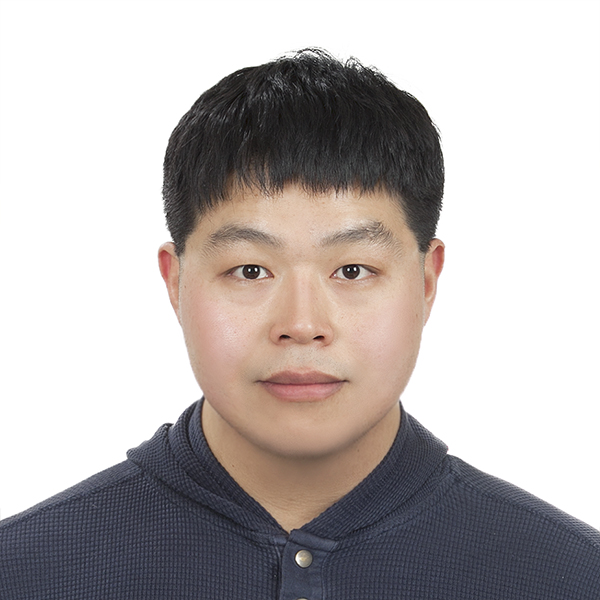Author

Heung-Sik Kim Ph.D | Assistant Professor, Department of Physics, Kangwon National University, Korea